# Oversimplified: Signals and Systems (3.5) – Prereq: Basic Math

I have saved a very concise primer written by Dr. Sebald who taught in University of Wisconsin – Madison after I have graduated: Basic Math for Signal Processing (Dr. Sebald)

There are 11 sections there and some of them I think it’s gold and some of them I think beginners can skip (but still good to know for mathematical maturity you’ll need later).

Section 1: Notations

I love his treatment of commonly used notation habits (Table 1) because there’s often a lot of variables to keep track of in signal processing math that it’d drive us insane if we and our readers have to remember what each variable means each time we start a new project.

Completing the square and solving quadratic equations is less important in general signal processing. The only time you’ll see it is factorizing in partial fractions, and the matrix form of completing squares in optimization related topics (such as Recursive Least Squares)

Section 3 and 7: Complex Numbers

Basically you have to recognize polar and rectangular coordinates and remember properties (a) to (i) by heart. It’s so important that I dedicated a page for it.

Section 4: Sequences and Series

Getting comfortable with geometric series is the most important thing you’ll need to know for DSP or Discrete Time Signal Processing

Section 5: Differentiation

You don’t need a lot of fancy Calculus in signal processing. You only need the simple stuff. I’ve never used a quotient rule and L’Hopital rule in signal processing for sure and I barely remember using product rule. Never used differentials at all.

The most important thing you need to know is that calculus operations are LINEAR operators so all superposition tricks applies.

Differentiability and continuity (i.e. smoothness) is an important qualitative concept when we get to the transforms as they represents fast changes that will be spread out all over the place in another domain.

Section 6: Integration

It has a little more rigorous treatment to Riemann integrals we learn in calculus. Most of the time you don’t need to dive into such details unless you run into theoretical understanding of transforms and generalized functions (also called distributions, not the probability kind), and it’s a one-off thing so you appreciate the math of how the transform pairs are derived. After that you just use the transform pairs remembered like a pro.

We barely get to use fundamental theorem of calculus other than a one-off torture section where you plug-and-chug discontinuous functions into Fourier integrals. Basically after that ‘torture’ so you’ll appreciate how your transform tables are derived.

Unless you are deriving transform pairs that cannot be easily synthesized from the ones you already know, you should be only using the Fourier integral once in a blue moon or in a few cases where you can read the answer straight from the definition without much more work.

You are almost always on the wrong track doing things in a dumb way if you have to use integration by parts for signal processing questions.

Section 8: Taylor series

Series decomposition is often ninja tricks reserved for rare, difficult problems. The only one time I needed to do a series expansion is in Robert Gray’s old qualifying exam question which actually stems from generating function in probability, which is a z-transform: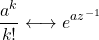Section 10: Mathematical Induction

You absolutely don’t need to know mathematical induction at all in signal processing. Sebald only quoted that to prove geometric series, which in my opinion is clumsy because we could have derived it like this: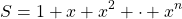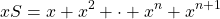Subtract the second expansion from the first so only the first termin the first equation and the last term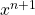in the second equation survives. Everything in between is canceled subtractively.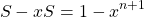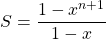Section 11: Logic

It’s just common sense that anybody working with math should know to think straight. It’s not directly used anywhere in basic signal processing classes. But I’d be concerned if you can survive any kind of engineering if you don’t at least know it.